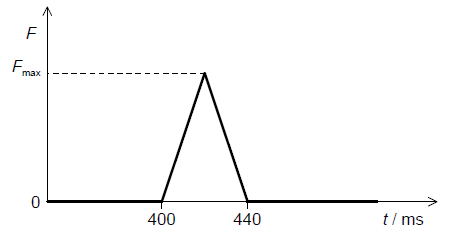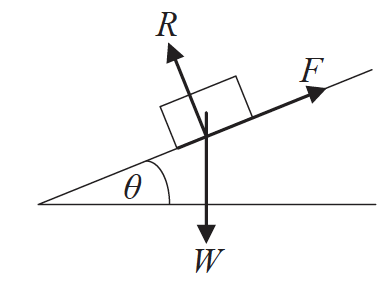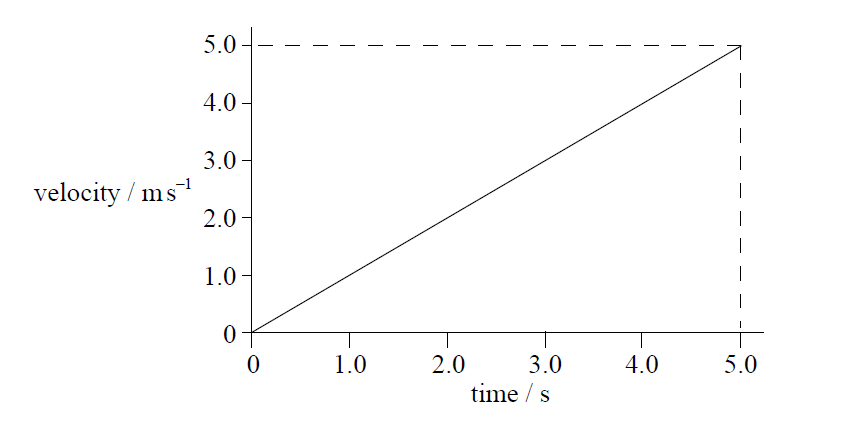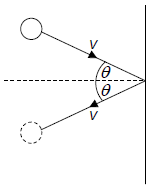1. An object is released from a stationary hot air balloon at height h above the ground.

An identical object is released at height h above the ground from another balloon that is rising at constant speed. Air resistance is negligible. What does not increase for the object released from the rising balloon?

2. A box is accelerated to the right across rough ground by a horizontal force

Fa. The force of friction is Ff. The weight of the box is Fg and the normal reaction is Fn. Which is the free-body diagram for this situation?

3. Which of the following is a correct statement of Newton’s second law of motion?
4. A ball is tossed vertically upwards with a speed of

5.0 m s–1. After how many seconds will the ball return to its initial position?

5. Three coplanar forces of 5 N, 6 N and 7 N act on an object. Which force could not be the resultant of these three forces?
6. A motor of input power 160 W raises a mass of 8.0 kg vertically at a constant speed of 0.50

m s–1.

What is the efficiency of the system?

7. A cart of mass 4.0 kg is being pulled with a force of 24 N. The cart accelerates at 3.0

m s–2. What is the net force on the cart?

8. In an inelastic collision
9. Two boxes in contact are pushed along a floor with a force F. The boxes move at a constant speed. Box X has a mass m and box Y has a mass 2m.What is the resultant force acting on Y?

10. The graph shows how the velocity of a particle varies with time.Which of the following graphs correctly shows how the acceleration of the particle varies with time?

11. The net force on a body is F. The impulse of F is equal to the
12. A skydiver of mass 80 kg falls vertically with a constant speed of 50

m s−1. The upward force acting on the skydiver is approximately

13. The efficiency of an electric motor is 20 %. When lifting a body 500 J of energy are wasted. What is the useful work done by the motor?
14. A spring of negligible mass and length l0 hangs from a fixed point. When a mass m is attached to the free end of the spring, the length of the spring increases to l. The tension in the spring is equal to k∆x, where k is a constant and ∆x is the extension of the spring. What is k?
15. A ball of weight W is travelling horizontally towards a vertical wall. It strikes the wall and rebounds horizontally. The change in the magnitude of the momentum of the ball is Δ p . Which of the following is the magnitude of the impulse that the ball imparts to the wall?16. A weight W is tied to a trolley of mass M by a light string passing over a frictionless pulley. The trolley has an acceleration a on a frictionless table. The acceleration due to gravity is g.What is ?

17. An inelastic collision occurs between two bodies in the absence of external forces.

What must be true about the total momentum of the two bodies and the total kinetic energy of the two bodies during this interaction?

18. A ball of mass 0.2 kg strikes a force sensor and sticks to it. Just before impact the ball is travelling horizontally at a speed of 4.0

m s–1. The graph shows the variation with time t of the force F recorded by the sensor.What is Fmax?

19. An object of weight W is falling vertically at a constant speed in a fluid. What is the magnitude of the drag force acting on the object?
20. A stone is falling at a constant velocity vertically down a tube filled with oil. Which of the following statements about the energy changes of the stone during its motion are correct?

I.     The gain in kinetic energy is less than the loss in gravitational potential energy.

II.    The sum of kinetic and gravitational potential energy of the stone is constant.

III.   The work done by the force of gravity has the same magnitude as the work done by friction.

21. A tennis ball is released from rest at a height h above the ground. At each bounce 50 % of its kinetic energy is lost to its surroundings. What is the height reached by the ball after its second bounce?
22. A block of weight W slides down an inclined plane at a constant speed.The normal reaction acting between the block and the plane is R and the frictional force between the block and the plane is F. The incline is at an angle θ to the horizontal. What is the magnitude of F?

23. The velocity–time graph for an accelerating object that is traveling in a straight line is shown below.Which of the following is the change in displacement of the object in the first 5.0 seconds?

24. A brother and sister take the same time to run up a set of steps. The sister has a greater mass than her brother. Which of the following is correct?25. The variation of the displacement of an object with time is shown on a graph. What does the area under the graph represent?
26. A ball of mass m strikes a vertical wall with a speed v at an angle of θ to the wall. The ball rebounds at the same speed and angle. What is the change in the magnitude of the momentum of the ball?27. An object rotates in a horizontal circle when acted on by a centripetal force F. What is the centripetal force acting on the object when the radius of the circle doubles and the kinetic energy of the object halves?
28. A heat engine does 300 J of work during one cycle. In this cycle 900 J of energy is wasted. What is the efficiency of the engine?
29. A student of weight 600N climbs a vertical ladder 6.0m tall in a time of 8.0s. What is the power developed by the student against gravity?
30. In the collision between two bodies, Newton’s third law
31. A small positively charged sphere is suspended from a thread and placed close to a negatively charged rod. When the thread is at 45° to the vertical the system is in equilibrium. The weight of the sphere is W and the magnitude of the electrostatic force between the rod and the sphere is F.What is the magnitude of compared with the magnitude of ?

32. A body moves in a straight line. In order for the equations for uniformly accelerated motion to be applied, which condition must be true?
33. A skydiver jumped out of an airplane. On reaching a terminal speed of

60 ms−1, she opened her parachute. Which of the following describes her motion after opening her parachute?

34. An insect of mass m jumps vertically from rest to a height h. The insect releases the energy needed for the jump in time Δt. What is the estimate for the power developed by the insect?
35. A nuclear power station produces 10 GW of electrical power. The power generated by the nuclear reactions in the core of the reactor is 25 GW. The efficiency of the power station is
36. A raindrop falling from rest at time t = 0 reaches terminal velocity. Which graph best represents how the speed v varies with time t ?
37. A ball falls vertically and bounces off the ground. Immediately before impact with the ground the speed of the ball is u . Immediately after leaving the ground the speed is v .Which of the following expressions is the ratio of38. A wooden block is sliding down an inclined plane at constant speed. The magnitude of the frictional force between the block and the plane is equal to
39. A driving force F acts on a car which moves with constant velocity v. The quantity Fv is equivalent to the
40. An object is moving in a straight line. A force F and a resistive force f act on the object along the straight line.Both forces act for a time t.

What is the rate of change of momentum with time of the object during time ?

41. A ball of mass m is thrown with an initial speed of u at an angle θ to the horizontal as shown. Q is the highest point of the motion. Air resistance is negligible.What is the momentum of the ball at Q?

42. The distances between successive positions of a moving car, measured at equal time intervals, are shown.The car moves with

43. A uniform ladder resting in equilibrium on rough ground leans against a smooth wall. Which diagram correctly shows the forces acting on the ladder?
44. An increasing force acts on a metal wire and the wire extends from an initial length

/0 to a new length /. The graph shows the variation of force with length for the wire. The energy required to extend the wire from /0 to / is E.The wire then contracts to half its original extension.

What is the work done by the wire as it contracts?

45. An object of mass m is initially at rest. When an impulse I acts on the object its final kinetic energy is

EK. What is the final kinetic energy when an impulse of 2I acts on an object of mass 2m initially at rest?